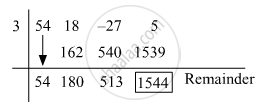Advertisement Remove all ads

# Divide the First Polynomial by the Second Polynomial and Find the Remainder Using Factor Theorem . - Algebra

Sum

Divide the first polynomial by the second polynomial and find the remainder using factor theorem .

(54m^3 + 18 m^2 - 27m + 5) ; (m - 3)

Advertisement Remove all ads

#### Solution

By synthetic division:

Dividend = (54m^3 + 18 m^2 - 27m + 5)

Divisor = m − 3

Opposite of −3 = 3The coefficient form of the quotient is (54, 180, 513).

∴ Quotient = 54x2 + 180x + 513

Remainder = 1544

By remainder theorem:

Let p(m) = 54m^3 + 18m^2 - 27m + 5

Divisor = m − 3

By remainder theorem,

Remainder = p(3)

= 54 xx (3)^3 + 18 xx (3)^3 - 27 xx 3 + 5
= 54 xx 27 + 18 xx 9 - 27 xx 3 + 5
= 1458 + 162 - 81 + 5
= 1544

Is there an error in this question or solution?
Advertisement Remove all ads

#### APPEARS IN

Balbharati Mathematics 1 Algebra 9th Standard Maharashtra State Board
Chapter 3 Polynomials
Practice Set 3.5 | Q 5.3 | Page 53
Advertisement Remove all ads
Advertisement Remove all ads
Share
Notifications

View all notifications

Forgot password?